# Measuring the Melt Density of a Polymer using a Melt Indexer

Method A/B is a test that can be performed in a melt flow rate tester so as to calculate the value of the polymer’s melt density at a certain temperature. Both a “method A” test and a “method B” test are performed on the same charge of material in a method A/B test.

The melt flow rate from method A is equated to the method B flow rate equation and solved for apparent melt density. This test is valuable because it means that a valid apparent melt density can be obtained. This can be used later in a solo method B test (in method B there are no manual cuts of extrudate or weighing) in order to achieve results that are equivalent to method A (in method A the operator has to manually weigh and cut the sample).

The mass melt flow rate from Method A is calculated using the mass of the extrudate(s), as shown in the equation below: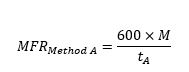where (g) is the mass in grams of material, collected over time (sec). In order to convert the unit of MFR to g/10 min the value “600” is used.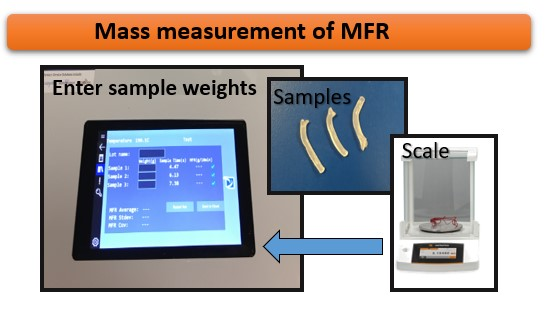Volumetric displacement measurement of MVR using a Dynisco LMI5500. Image Credit: Dynisco

The equation below demonstrates how the melt volume flow rate (MVR) from Method B is calculated: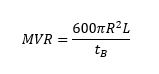where R is standard piston radius (R = 0.477 cm), tB is the time of the piston travel for distance of L. Based on ASTM D1238, L can be set to be 0.635 ± 0.025 cm or 2.54 ± 0.025 cm measuring range depending in the expected MFR of the samples.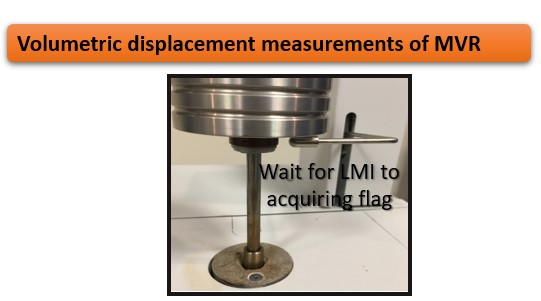Volumetric displacement measurement of MVR using a Dynisco LMI5500. Image Credit: Dynisco

The ratio of the two values (MFR from Method A and MVR from Method B) is a measure of the melt density of the polymer in g/cm3 and is shown in the equation below: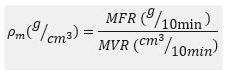The results for apparent melt density for two different EVA materials, using a Dynisco LMI series melt indexer (weight: 2.16 kg, test temperature: 190 oC) are shown in Figure 1. For the Method A part of this test, 3 cuts were collected within a 1 minute time interval. For the Method B part, 3 flags were acquired with a flag length of 6.35 mm.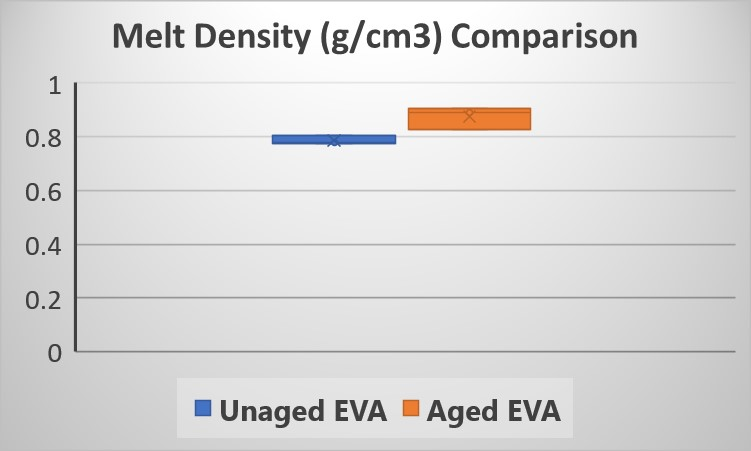Figure 1. Apparent melt density results from Dynisco LMI series. Image Credit: Dynisco

This apparent melt density definition forces the two test methods to agree. It is termed the “apparent” melt density because it is actually a correlation coefficient which forces Method A and Method B to agree. A true melt density could only be assessed if the extrudate was bubble-free, there was no leakage past the plunger tip, and a few other minor factors were taken into consideration.

This apparent melt density value can be used to obtain MFR values in Method B tests without manually weighing and cutting the samples. It is important to note that the melt density of the polymer is a function of temperature. The density of the material also tends to be changed by reinforcing agents, fillers, etc.

## Acknowledgments

Produced from materials originally authored by Azadeh Farahanchi, Rheological Scientist, Ph.D.This information has been sourced, reviewed and adapted from materials provided by Dynisco.

## Citations

• APA

Dynisco. (2020, April 27). Measuring the Melt Density of a Polymer using a Melt Indexer. AZoM. Retrieved on October 24, 2020 from https://www.azom.com/article.aspx?ArticleID=19176.

• MLA

Dynisco. "Measuring the Melt Density of a Polymer using a Melt Indexer". AZoM. 24 October 2020. <https://www.azom.com/article.aspx?ArticleID=19176>.

• Chicago

Dynisco. "Measuring the Melt Density of a Polymer using a Melt Indexer". AZoM. https://www.azom.com/article.aspx?ArticleID=19176. (accessed October 24, 2020).

• Harvard

Dynisco. 2020. Measuring the Melt Density of a Polymer using a Melt Indexer. AZoM, viewed 24 October 2020, https://www.azom.com/article.aspx?ArticleID=19176.

## Tell Us What You Think